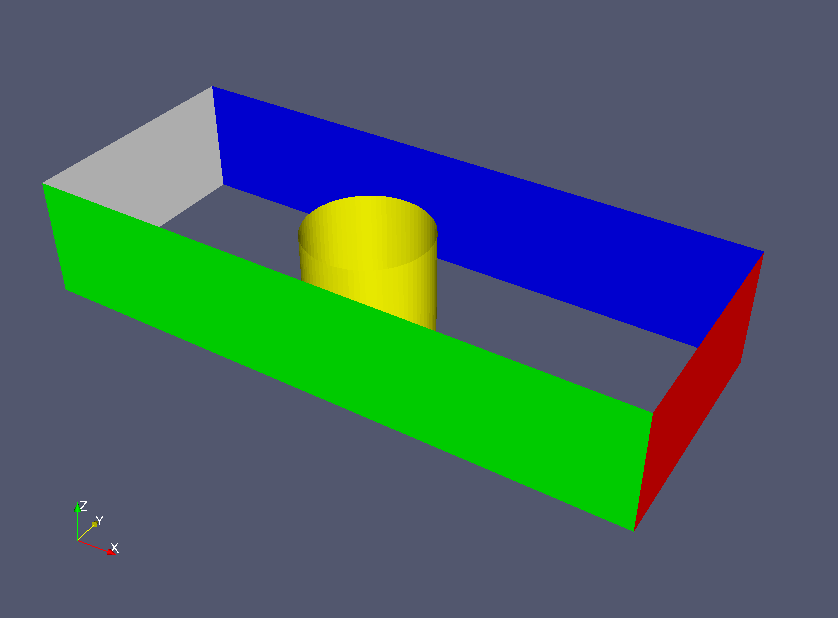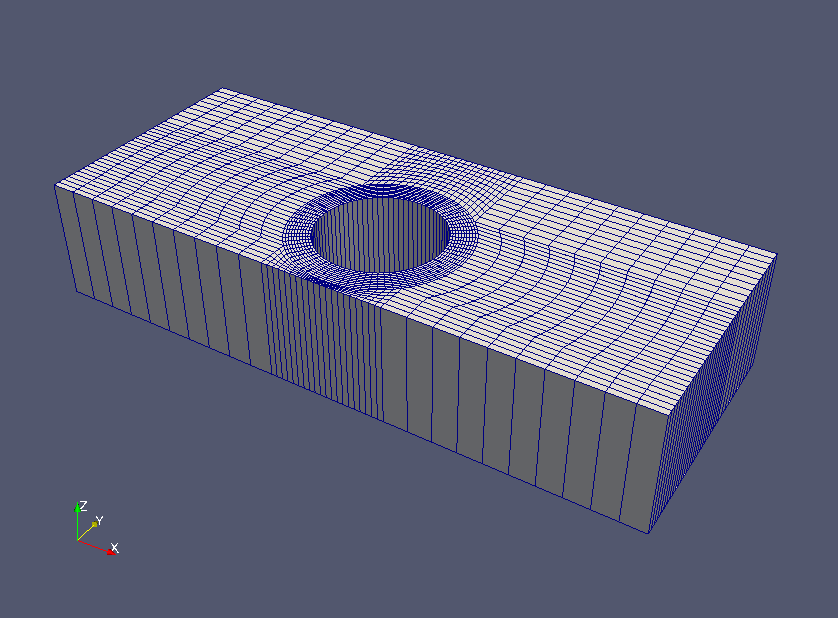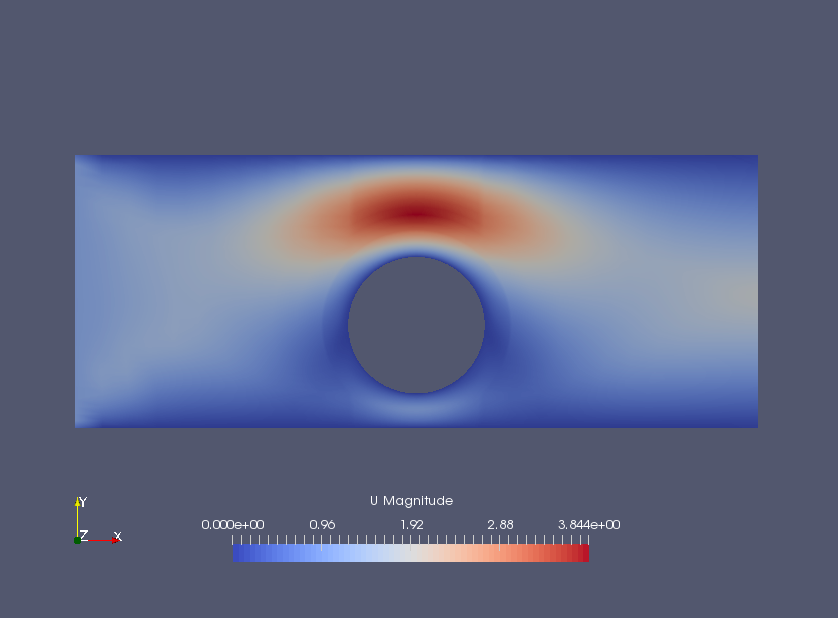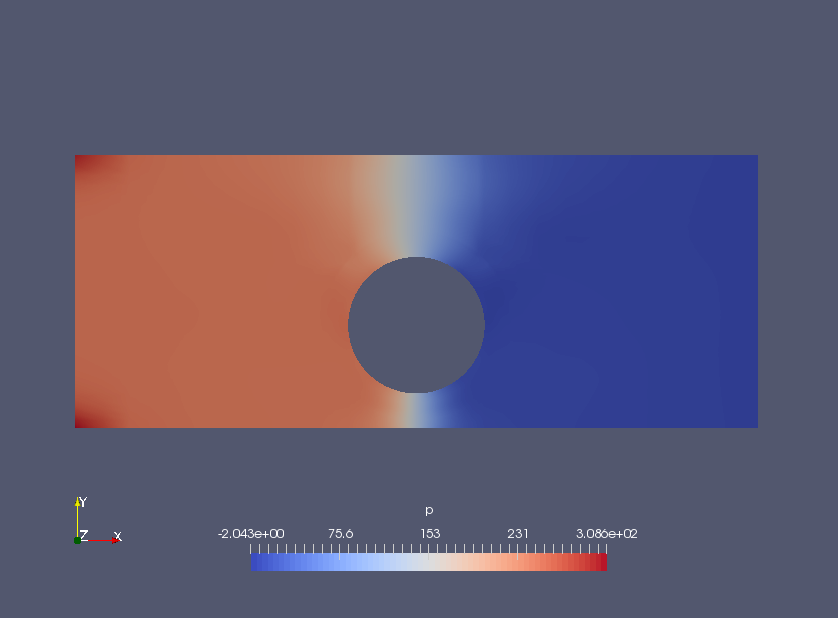﻿ Non-newtonian fluid flow around a cylinder - XSim

# Non-newtonian fluid flow around a cylinder

Update: June 1, 2017
OpenFOAM 4.x

$FOAM_TUTORIALS/incompressible/nonNewtonianIcoFoam/offsetCylinder ## Summary We calculate the flow of a non-Newtonian fluid in a channel with an obstacle on one side, up to 2 seconds later. The fluid flows in from the region "left" (white part) at a velocity of 1 m/s and flows out from the region "right" (red part). No-slip conditions are set for the cylinder and channel walls, and the flow is solved as 2-dimensional with a single mesh in the Z direction.Model geometry The meshes are as follows.Meshes The strain rateand the viscosity coefficient ν of the non-Newtonian fluid have the relationship as follows.The value of each parameter is defined in the file "constant/transportProperties" as follows. transportModel CrossPowerLaw; CrossPowerLawCoeffs { nu0 [0 2 -1 0 0 0 0] 0.01; nuInf [0 2 -1 0 0 0 0] 10; m [0 0 1 0 0 0 0] 0.4; n [0 0 0 0 0 0 0] 3; }  The calculation result is as follows.Flow velocity (U)Pressure (p) If it is a Newtonian fluid, the flow velocity will be higher in the narrower channel, but since it is a non-Newtonian fluid, the flow velocity will be higher in the wider channel. ## Commands cp -r$FOAM_TUTORIALS/incompressible/nonNewtonianIcoFoam/offsetCylinder offsetCylinder
cd offsetCylinder

blockMesh
nonNewtonianIcoFoam

paraFoam

## Calculation time

--- *Single, Core(TM) i7-2600 CPU @ 3.40GHz 3.40GHz